Start your cart here complete your order on Maillot-Erable or Japon-Import and reduce your shipping costs##### The Japanese Bonsai specialist
Direct order Contact Help / Services Newsletter# Stone lantern kanjuji 120 cm

Kanjuji lanterne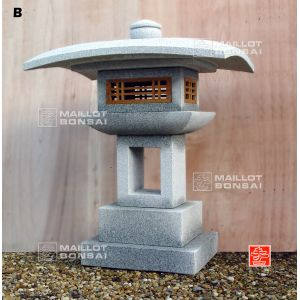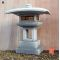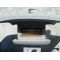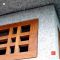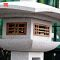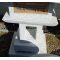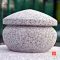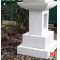ref. : 7430980,00

voluminous/heavy item extra shipping of 65,00

Available quantity : 2Order

###### Description

Height 120 cm. Width of the top section +- 90*75 cm, weight approximately 365 kilograms, delivered in 7 elements. A Japanese lantern of neat proportions made of solid granite and featuring a wide wooden screen window. A lamp can be added to this model to light the garden by night.

#lantern 5.7 #stone 4.5 #kanjuji 2.7 #approximately 2.3 #proportions 2.1 #kilograms 1.9 #delivered 1.9 #featuring 1.9 #elements 1.8 #japanese 1.8

Formule
(( ROUND((CHAR_LENGTH(b.article_nom)-CHAR_LENGTH(REPLACE(b.article_nom, 'lantern', '')))/LENGTH('lantern')) + ROUND((CHAR_LENGTH(b.article_description)-CHAR_LENGTH(REPLACE(b.article_description, 'lantern', '')))/LENGTH('lantern')) ) * 3.7) + (( ROUND((CHAR_LENGTH(b.article_nom)-CHAR_LENGTH(REPLACE(b.article_nom, 'kanjuji', '')))/LENGTH('kanjuji')) + ROUND((CHAR_LENGTH(b.article_description)-CHAR_LENGTH(REPLACE(b.article_description, 'kanjuji', '')))/LENGTH('kanjuji')) ) * 2.7) + (( ROUND((CHAR_LENGTH(b.article_nom)-CHAR_LENGTH(REPLACE(b.article_nom, 'stone', '')))/LENGTH('stone')) + ROUND((CHAR_LENGTH(b.article_description)-CHAR_LENGTH(REPLACE(b.article_description, 'stone', '')))/LENGTH('stone')) ) * 2.5) + (( ROUND((CHAR_LENGTH(b.article_nom)-CHAR_LENGTH(REPLACE(b.article_nom, 'approximately', '')))/LENGTH('approximately')) + ROUND((CHAR_LENGTH(b.article_description)-CHAR_LENGTH(REPLACE(b.article_description, 'approximately', '')))/LENGTH('approximately')) ) * 2.3) + (( ROUND((CHAR_LENGTH(b.article_nom)-CHAR_LENGTH(REPLACE(b.article_nom, 'proportions', '')))/LENGTH('proportions')) + ROUND((CHAR_LENGTH(b.article_description)-CHAR_LENGTH(REPLACE(b.article_description, 'proportions', '')))/LENGTH('proportions')) ) * 2.1) + (( ROUND((CHAR_LENGTH(b.article_nom)-CHAR_LENGTH(REPLACE(b.article_nom, 'kilograms', '')))/LENGTH('kilograms')) + ROUND((CHAR_LENGTH(b.article_description)-CHAR_LENGTH(REPLACE(b.article_description, 'kilograms', '')))/LENGTH('kilograms')) ) * 1.9) + (( ROUND((CHAR_LENGTH(b.article_nom)-CHAR_LENGTH(REPLACE(b.article_nom, 'delivered', '')))/LENGTH('delivered')) + ROUND((CHAR_LENGTH(b.article_description)-CHAR_LENGTH(REPLACE(b.article_description, 'delivered', '')))/LENGTH('delivered')) ) * 1.9) + (( ROUND((CHAR_LENGTH(b.article_nom)-CHAR_LENGTH(REPLACE(b.article_nom, 'featuring', '')))/LENGTH('featuring')) + ROUND((CHAR_LENGTH(b.article_description)-CHAR_LENGTH(REPLACE(b.article_description, 'featuring', '')))/LENGTH('featuring')) ) * 1.9) + (( ROUND((CHAR_LENGTH(b.article_nom)-CHAR_LENGTH(REPLACE(b.article_nom, 'elements', '')))/LENGTH('elements')) + ROUND((CHAR_LENGTH(b.article_description)-CHAR_LENGTH(REPLACE(b.article_description, 'elements', '')))/LENGTH('elements')) ) * 1.8) + (( ROUND((CHAR_LENGTH(b.article_nom)-CHAR_LENGTH(REPLACE(b.article_nom, 'japanese', '')))/LENGTH('japanese')) + ROUND((CHAR_LENGTH(b.article_description)-CHAR_LENGTH(REPLACE(b.article_description, 'japanese', '')))/LENGTH('japanese')) ) * 1.8)

## Secure payment## Delivery

Our logistic partners :## Advice, Orders

04 74 55 23 48
Pépinière MAILLOT-BONSAÏ
Le Bois Frazy
01990 RELEVANT - FRANCE
on appointment
My account My cart Newsletter RSS subscription Help / ServicesThe Japanese Bonsai specialist
If you like Maillot Bonsaï, share on  facebook

Pensez à venir nous rendre visite sur rendez vous.

Guy Maillot

OK